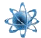xProbability distribution
Posts  1 - 1  of  1syedamer
Hi,
I am working on finding out probability density function of a random vector(state vector of position and velocity) in terms of gaussian probability multiplied by hermite polynomials. The problem is how to find out coefficients of such series and how to find their rates(differentials).If any body could help me out.